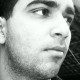# Cups and BallsGISYERLOOT FAIR had arrived in town .  “Roll up! Roll up!” shouted one stall owner . “A prize for guessing the number of balls under the cups!”

On the counter in front of him were four upturned cups. Each concealed the same number of balls . On each cup was a statement about the number of balls underneath. From left to right they read as follows.

“One or four,” “Two or four,”  “Two or three,” or “One or two,” . Only one of the four statements was correct .

How many balls were under a cup and which statement was true ?

### See our previous ‘Puzzles of the Day’:

1.3.. statement two or three is true.

1.How the ans is three can you plz explain

2.Three(3) balls
Statement on cup 3: Two or three is true

3.3
Statement two or three is correct

4.The statement 3 is correct.The number of balls are 3

5.Prateek Trehan

Assuming statement one is not a typo. It is the true statement and there is one ball underneath each cup

6.one ball
statement 4

7.Manish Jindal

3rd from left: “Two or three”

8.3

• What is the solution?

By Akhilesh Poddar, 4 weeks ago

• In the island of Hoola Boola Moola , inhabitants have a...

By Sanket211, 3 months ago

• For a=0.9086, x= 1.0286 and a= -1.6627, x= 4.9173

By Aditya Dang, 4 months ago

• Since, x+1 satisfies the equation ax^2+ bx+ c=0. Theref...

By Aditya Dang, 4 months ago

• The HCF of 141 and 517 is 47. But 47 does not completel...

By Aditya Dang, 4 months ago

• 1. How many integral pairs (x, y), where 0< x, y <...

By lastknight99, 4 months ago

• Hi, It is given that a= (212)b . Forming an equation...

By Aditya Dang, 4 months ago

• Perimeter of circle is 2pi(793)^1/2.

By Aditya Dang, 4 months ago

• Area of ΔDOC is 21cm^2.

By Aditya Dang, 4 months ago

• Length of smaller of two sides is equal to 36 units.

By Aditya Dang, 4 months ago

• Length of AB is (17)^1/2 units.

By Aditya Dang, 4 months ago

• The sum borrowed is Rs. 3300.

By Aditya Dang, 4 months ago